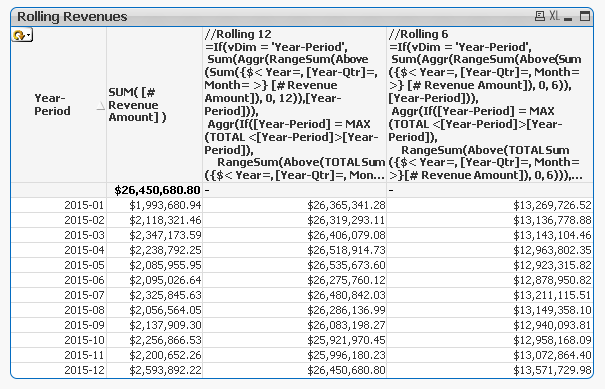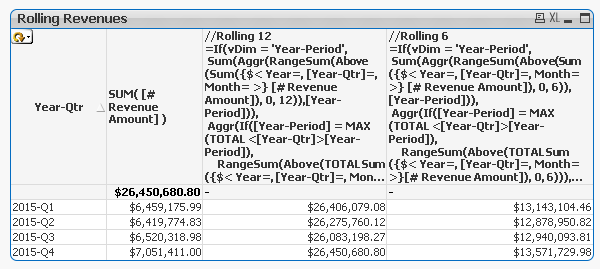# QlikView App Dev

Discussion Board for collaboration related to QlikView App Development.

Announcements
cancel
Showing results for
Did you mean:Not applicable

## Rolling N Period Revenues with RangeSum, Above, & AGGR

I have an app with revenues by month (lowest level of granularity). Need to calculate rolling N periods on the fly, when the chart dimension is allowed to change with a cyclic group. The cyclic group "Periods" goes through 3 levels of reporting granularity:  `Year-Period` -> 'Year-Qtr` -> `Year`.

The following formula works like a charm for the most granular setting (i.e. `Year-Period`):

```SUM(AGGR(RangeSum(Above(Sum(     {\$< Year=, [Year-Qtr]=, Month= >}[# Revenue Amount]   ),0,\$1)),[Year-Period]  ) )
```

where \$1 is set to 12 for rolling 12, 6 for rolling 6, etc.

However, whenever the chart is cycled to `Year-Qtr` or `Year`, the calculations are incorrect, as the formula will aggregate 6 years above for \$1 = 6 with Year selected, etc. I've considered some sort of workarounds such as dividing the \$1 value by a factor based on the value of the cyclic group dimension (using `GetCurrentField(Periods)` in a conditional formula to provide a divisor for the N value passed into the parameter). However, this seems a bit clunky and presents some problems - for instance, if we want Rolling 6 months and the user selects `Year`, we'll get 0.5 as the row count in the RangeSum expression.

I've attached a sample QVD. I've been trying to crack this Rolling N calculation a number of ways, including with an AsOf Dates table...but I keep running into a wall whenever the granularity of the chart dimension changes.

Appreciate any inputs! Thank you.

1 Solution

Accepted SolutionsMVP

Try these two expressions out:

//Rolling 12

=If(vDim = 'Year-Period',

Sum(Aggr(RangeSum(Above(Sum({\$< Year=, [Year-Qtr]=, Month= >} [# Revenue Amount]), 0, 12)),[Year-Period])),

Aggr(If([Year-Period] = MAX(TOTAL <\$(=vDim)>[Year-Period]),

RangeSum(Above(TOTAL Sum({\$< Year=, [Year-Qtr]=, Month= >}[# Revenue Amount]), 0, 12))),

\$(=vDim), [Year-Period]))

//Rolling 6

=If(vDim = 'Year-Period',

Sum(Aggr(RangeSum(Above(Sum({\$< Year=, [Year-Qtr]=, Month= >} [# Revenue Amount]), 0, 6)),[Year-Period])),

Aggr(If([Year-Period] = MAX(TOTAL <\$(=vDim)>[Year-Period]),

RangeSum(Above(TOTAL Sum({\$< Year=, [Year-Qtr]=, Month= >}[# Revenue Amount]), 0, 6))),

\$(=vDim), [Year-Period]))

Where vDim = ='[' & GetCurrentField(Periods) & ']'

When on Year-Period:When on Year:When on Year-Qtr:3 RepliesMVP

What is the output that you are hoping to see?Not applicable
Author

I want the formula to return the correct rolling N-period amount regardless of what dimension the user selects. If it's a rolling 6-month, then I want it to show the sum of the prior 6 months of revenue as of the dimension date. If the user has selected Year in the cyclic dimension, then the chart should show rolling 6 months as of the end of the year (i.e. 12/31/20XX). If the user selects Year-Quarter in the cyclic dimension, the chart would show the sum of revenues for the past six months - i.e. sum of the current and prior quarters for each dimension value.

Does that make sense?MVP

Try these two expressions out:

//Rolling 12

=If(vDim = 'Year-Period',

Sum(Aggr(RangeSum(Above(Sum({\$< Year=, [Year-Qtr]=, Month= >} [# Revenue Amount]), 0, 12)),[Year-Period])),

Aggr(If([Year-Period] = MAX(TOTAL <\$(=vDim)>[Year-Period]),

RangeSum(Above(TOTAL Sum({\$< Year=, [Year-Qtr]=, Month= >}[# Revenue Amount]), 0, 12))),

\$(=vDim), [Year-Period]))

//Rolling 6

=If(vDim = 'Year-Period',

Sum(Aggr(RangeSum(Above(Sum({\$< Year=, [Year-Qtr]=, Month= >} [# Revenue Amount]), 0, 6)),[Year-Period])),

Aggr(If([Year-Period] = MAX(TOTAL <\$(=vDim)>[Year-Period]),

RangeSum(Above(TOTAL Sum({\$< Year=, [Year-Qtr]=, Month= >}[# Revenue Amount]), 0, 6))),

\$(=vDim), [Year-Period]))

Where vDim = ='[' & GetCurrentField(Periods) & ']'

When on Year-Period:When on Year:When on Year-Qtr: